Chapter 11 The conservation of electric charge

The electric charge conservation is one of the basic conservation law in physics.

The electric charge conservation law is as follows:(11.1)

In which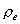is the electric charge density.

Let us prove that the electric charge conservation equation is valid for the electron.

Combine the electron velocity equation (10.11) and the electric field equation (4.2), then we can get:(11.2)

As we know: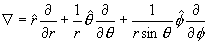(11.3)

Thus: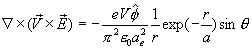(11.4)

From the electric charge density equation (2.1), thus:(11.5)

From equation (4.2), we know that:(11.6)

Thus, we can get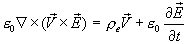(11.7)

Compare this with the hydromagnetic equation (dynamo equation), which is as follows: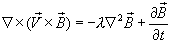(11.8)

In whichis the constant of the magnetic diffusivity.

We can see that there are many similarities between these two equations; we then call the equation  (11.7) as the hydroelectric equation of electromagnetic field.

Because:(11.9)

Thus: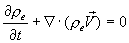(11.10)

Equation (11.10) is the electron electric charge conservation equation, which is also called the electric charge continuity equation.